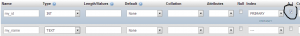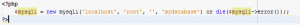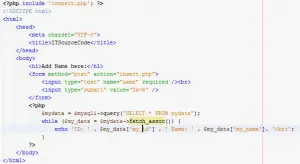Simple Insert and Show Data from Database in PHP and MYSQL

INSERTING AND SHOWING DATA FROM DATABASE IN PHP & MYSQL

Good day everyone! Today I am going to teach you some basic knowledge about on how to insert data in database in PHP as well as showing the data inserted on it. This tutorial has a big help for beginners for starting up their dynamic websites.

Ok! Let’s start our tutorial.

First, create an “index.php” file then put the following codes.

[php]

<html>

<title>ITSourcecode</title>

<body>

<form method=”post” action=”insert.php”>

<input type=”text”  name=”name” /><br>

<input type=”submit” value=”Save” />

</form>

</body>

</html>

[/php]

Output:Second, create a database. Name it as “mydatabase”.

Then create table, name it as “mydata”, then put the following columns:

my_id = primary keymy_name = textJust like this:Then create a “connect.php” file then insert the following codes.Then create an “insert.php” file, then put the following codes.

[php]

<?php

Include ‘connect.php’;

if (isset(\$_POST[‘name’])) {

\$name = \$_POST[‘name’];

\$insert_name = \$mysqli->query(“INSERT INTO mydata (my_name) VALUES (‘\$name’)”);

if (\$insert_name) {

}

}

?>

[/php]

Now, try to insert data.Now, let’s show all the data inserted in the database.

On the index file, put the following codes below after the form.

[php]

<php

\$mydata = \$mysqli->query(“SELECT * FROM mydata”);

While (\$my_data = \$mydata->fetch_assoc()) {

Echo ‘ID: ’ . \$my_data[‘my_id’] . ‘Name: ’ . \$mydata[‘my_name’]. ‘<br>’;

}

?>

[/php]Output:Then try to insert another data.That’s all and thank you.

1 thought on “Simple Insert and Show Data from Database in PHP and MYSQL”

1.Hello